Select Page

# Maths Relations and Functions CBSE 12 Science MCQ in English Solutions

Maths Relations and Functions CBSE 12 Science MCQ in English Solutions to enable students to get Solutions in a narrative video format for the specific question.

Expert Teacher provides Maths Relations and Functions CBSE 12 Science MCQ English Solutions. This video solution will be useful for students to understand how to write an answer in exam in order to score more marks. This teacher uses a narrative style for a question from Relations and Functions not only to explain the proper method of answering question, but deriving right answer too.

Please find the question below and view the Solution in a narrative video format.

Question:

Solution Video in English:

You can select video Solutions from other languages also. Please check Solutions in ( Hindi )

## Similar Questions from CBSE, 12th Science, Maths, Relations and Functions

Question 1 : Let * be the binary operation on N given by a * b = LCM of a and b. Find 20 * 16. (View Answer Video)

Question 2 :  If f is the greatest integer function and g is the modulus function . Write the value of g o f(-1/3) – f o g ( -1/3 ) . (View Answer Video)

Question 3 : Let A ={1, 2, 3}. Then number of equivalence relations containing (1, 2) is: (View Answer Video)

Question 4 :  * is a binary operation on Z such that: a * b = a + b + ab. The solution of (3* 4) *x = – 1 is,

Question 5 : Consider a binary operation * on N defined as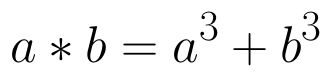. Choose the correct answer. (View Answer Video)

### Inverse Trigonometric Functions

Question 1 :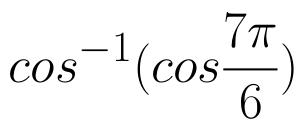is equal to : (View Answer Video)

Question 2 : Write the principal value of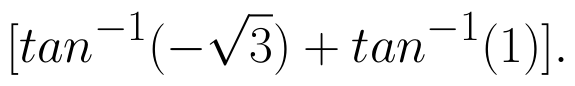(View Answer Video)

Question 3 : If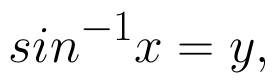then value of y lies between.....? (View Answer Video)

Question 4 : Write in the simplest form: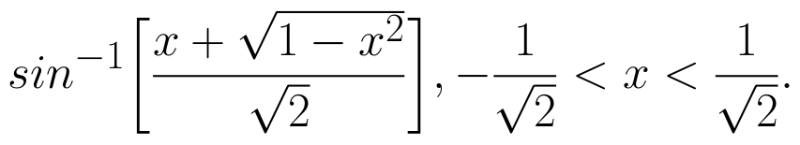(View Answer Video)

Question 5 : Solve for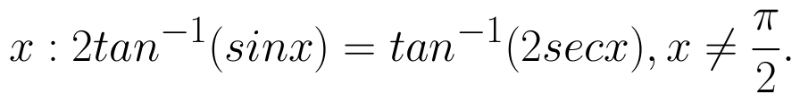(View Answer Video)

### Continuity and Differentiability

Question 1 : Differentiate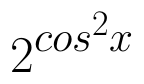w.r.t.x. (View Answer Video)

Question 2 : Differentiate w.r.t.x the function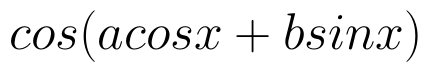, for some constant a and b. (View Answer Video)

Question 3 : Using the fact that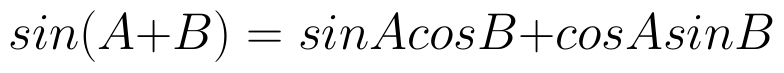and the differentiation, obtain the sum formula for cosines. (View Answer Video)

Question 4 : If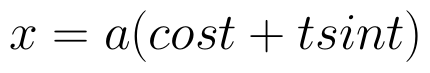and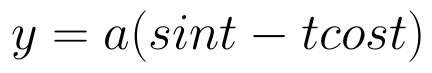, find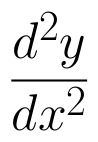. (View Answer Video)

Question 5 : Differentiate the function w.r.t.x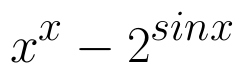. (View Answer Video)

### Application of Derivatives

Question 1 : Find the approximate change in the surface area of a cube of side x meters caused by decreasing the side by 1%. (View Answer Video)

Question 2 : If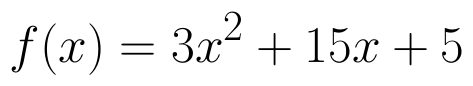, then the approximate value of f (3.02) is  (View Answer Video)

Question 3 : The line y = x + 1 is a tangent to the curve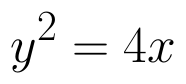at the point: (View Answer Video)

Question 4 : The line y=mx+1 is a tangent to the curve if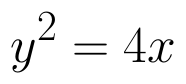the value of m is ________. (View Answer Video)

Question 5 : Find the approximate change in volume V of a cube of side x meters caused by increasing the side by 1%. (View Answer Video)